# NCERT Solutions for Class 10 Maths Exercise 13.4## myCBSEguide App

CBSE, NCERT, JEE Main, NEET-UG, NDA, Exam Papers, Question Bank, NCERT Solutions, Exemplars, Revision Notes, Free Videos, MCQ Tests & more.

NCERT solutions for Maths Surface Areas and Volumes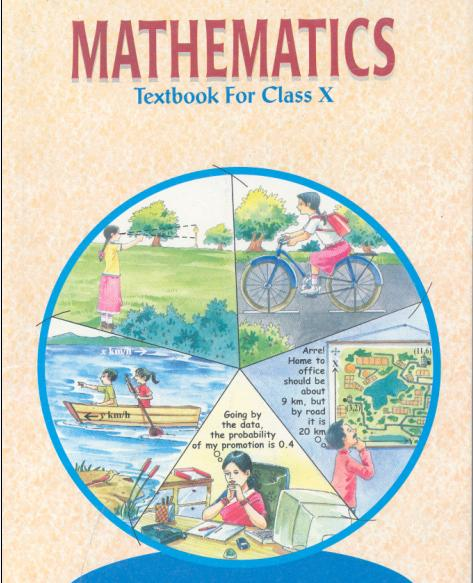## NCERT Solutions for Class 10 Maths Surface Areas and Volumes

###### 1. A drinking glass is in the shape of a frustum of a cone of height 14 cm. The diameters of its two circular ends are 4 cm and 2 cm. Find the capacity of the glass.

Ans. Here,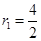= 2 m,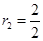= 1 m and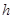= 14 m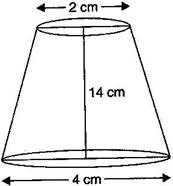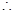Capacity of the glass =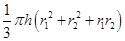=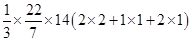=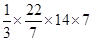=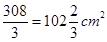NCERT Solutions for Class 10 Maths Exercise 13.4

###### 2. The slant height of a frustum of a cone is 4 cm and the perimeters (circumference) of its circular ends are 18 cm and 6 cm. Find the curved surface area of the frustum.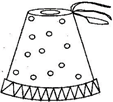Ans. Let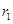cm and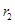cm be the radii of the ends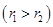of the frustum of the cone.

Then,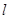= 4 cm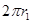= 18 cm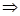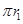= 9 cm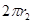= 6 cm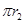= 3 cm

Now, CSA of the frustum =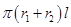=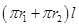=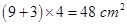NCERT Solutions for Class 10 Maths Exercise 13.4

###### 3. A fez, the cap used by the Turks, is shaped like the frustum of a cone (see figure). If its radius on the open side is 10 cm, radius at the upper base is 4 cm and its slant height is 15 cm, find the area of material used for making it.

Ans. Here,= 10 cm,= 4 cm and= 15 cmSurface area =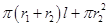=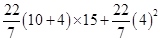=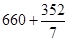=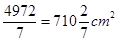NCERT Solutions for Class 10 Maths Exercise 13.4

###### 4. A container, opened from the top and made up of a metal sheet, is in the form of a frustum of a cone of height 16 cm with radii of its lower and upper ends as 8 cm and 20 cm respectively. Find the total cost of milk which can completely fill the container at the rate of Rs. 20 per liter. Also find the cost of metal sheet used to make the container, if it costs Rs. 8 per 100 cm2.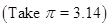Ans. Here,= 20 cm,= 8 cm and= 16 cmVolume of container ==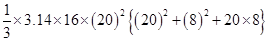=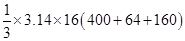=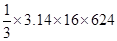=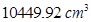= 10.44992 litersCost of the milk =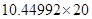= Rs. 208.8894 = Rs. 209

Now, surface area =+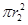=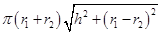+=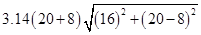+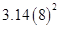=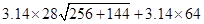= 1158.4 + 200.96

=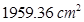Area of the metal sheet used =Cost of metal sheet =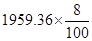= 156.7488 = Rs. 156.75

NCERT Solutions for Class 10 Maths Exercise 13.4

###### 5. A metallic right circular cone 20 cm high and whose vertical angle is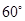is cut into two parts at the middle of its height by a plane parallel to its base. If the frustum so obtained be drawn into a wire of diameter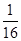cm, find the length of the wire.

Ans.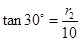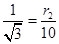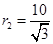cm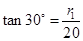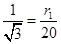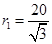cm= 10 cm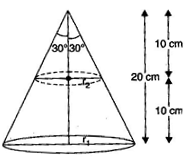Volume ==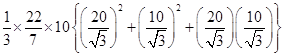=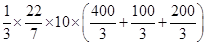=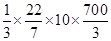=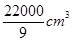Diameter of the wire =cmRadius of the wire =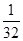cm

Let the length of the wire becm.

Then, Volume of the wire =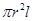=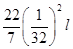=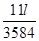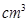According to the question,=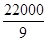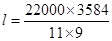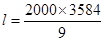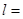796444.44 cm = 7964.4 m

## NCERT Solutions for Class 10 Maths Exercise 13.4

NCERT Solutions Class 10 Maths PDF (Download) Free from myCBSEguide app and myCBSEguide website. Ncert solution class 10 Maths includes text book solutions from Mathematics Book. NCERT Solutions for CBSE Class 10 Maths have total 15 chapters. 10 Maths NCERT Solutions in PDF for free Download on our website. Ncert Maths class 10 solutions PDF and Maths ncert class 10 PDF solutions with latest modifications and as per the latest CBSE syllabus are only available in myCBSEguide.

## CBSE app for Class 10

To download NCERT Solutions for Class 10 Maths, Computer Science, Home Science,Hindi ,English, Social Science do check myCBSEguide app or website. myCBSEguide provides sample papers with solution, test papers for chapter-wise practice, NCERT solutions, NCERT Exemplar solutions, quick revision notes for ready reference, CBSE guess papers and CBSE important question papers. Sample Paper all are made available through the best app for CBSE### 6 thoughts on “NCERT Solutions for Class 10 Maths Exercise 13.4”

1. So simple

2. right hai i had understand

3. Nice

4. Very easily understanding

5. Very workfull

6. It is very important to exam and easy to understand